# Calculated Fields

The Dashboard Designer supports calculated fields that allow you to apply complex expressions to data fields obtained from the dashboard’s data source. You can use these fields in data visualizations as regular data source fields.

Note

Calculated fields are not supported for the OLAP data source.

## Create a Calculated Field in the UI

After you have created a data source, you can add a new calculated field based on the existing data source fields.

To create a calculated field, select the required data source (and the required query/data member, if applicable) in the Data Source Browser and click the Add Calculated Field button in the Ribbon’s Data Source tab…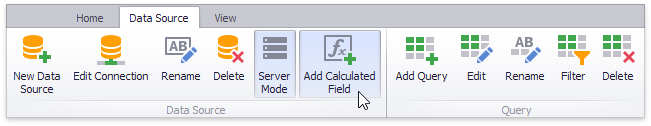…or right-click the Field List and select Add Calculated Field in the context menu.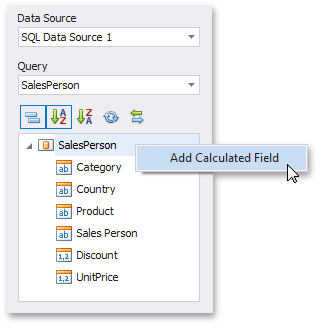This invokes the Expression Editor dialog, which allows you to specify an expression that will be used to obtain calculated field values. Here, you can construct the required expression.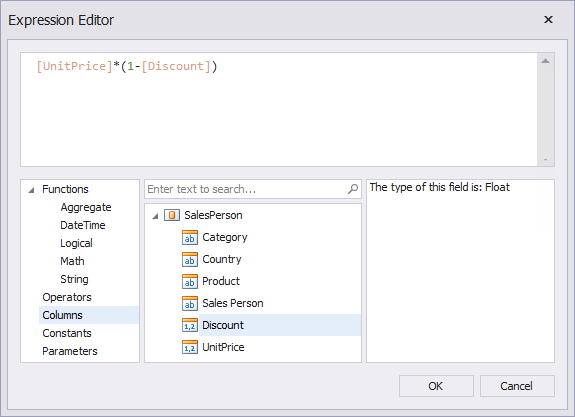You can use the following elements in expressions.

You can add a comment to your expression to explain it and make the expression more readable. Comments are multi-line and begin with `/*` and end with `*/`.

After the expression has been specified, click OK. This displays a new calculated field in the data source structure.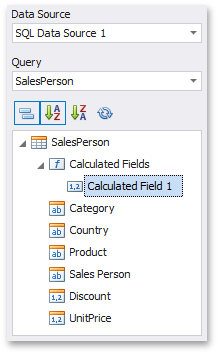You can now specify the calculated field type, change the default name, etc.

You can also pass dashboard parameters to a calculated field. See the following topic for details: Pass a Dashboard Parameter to a Calculated Field’s Expression.

## Edit a Calculated Field in the UI

To edit a calculated field, use its context menu.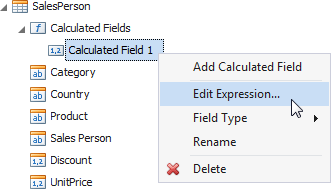This menu contains the following items:

Item

Description

Edit Expression…

Invokes the Expression Editor dialog, which allows you to change an expression for an existing calculated field.

Field Type

Specifies the type of the calculated field.

Note

Note that the type of a newly created calculated field is detected automatically based on the type of values returned by the specified expression. If you change this type, the expression will be wrapped to a corresponding conversion function (such as ToStr, ToDecimal, ToInt, etc.).

Rename

Changes the calculated field name.

Delete

Removes the existing calculated field from the data source.

## Create a Calculated Field in Code

Calculated fields are CalculatedField class instances. To create a new calculated field, pass the required settings to the CalculatedField constructor or use the following properties.

• Specify the data member of the calculated field. Assign a query to the CalculatedField.DataMember property (for DashboardSqlDataSource and DashboardEFDataSource). The SQL and EF Data Sources can contain multiple queries and the DataMember property of a calculated field determines to what query this field belongs.
• Specify the expression of the calculated field using the CalculatedField.Expression property.

Note

To specify the type of the calculated field, insert the required conversion function (such as ToStr, ToDecimal, ToInt, etc.) into the calculated field’s expression (the CalculatedField.Expression property). For instance, the following expression contains the ToDecimal conversion function that is used to change the default field type:

`ToDecimal([UnitPrice] * (1 - [Discount]))`

• Specify the name of the calculated field using the CalculatedField.Name property.

After the calculated field has been created, add it to the IDashboardDataSource.CalculatedFields collection of the data source’s calculated fields. Use the IDashboardDataSource.GetFieldSourceType property to get the calculated field’s type.

The following code snippet shows how to create a new calculated field based on the values of the ‘UnitPrice’ and ‘Discount’ data fields, and add it to the existing DashboardSqlDataSource.

``````using DevExpress.DashboardCommon;
//...

CalculatedField priceWithDiscount = new CalculatedField("[UnitPrice] * (1 - [Discount])");
priceWithDiscount.DataMember = "SalesPerson";
priceWithDiscount.Name = "Price with discount";